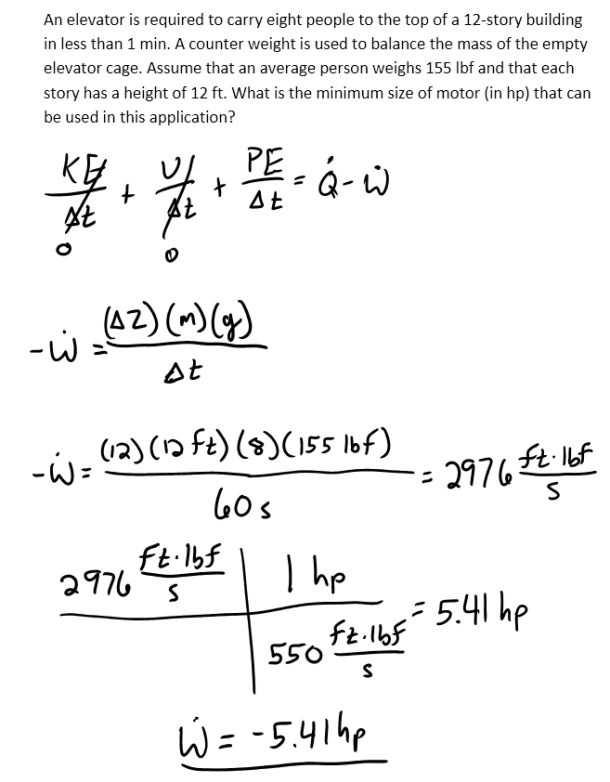heat and mass transfer problems engineering equations heat and mass transfer school homework engineering heat and mass transfer formulas heat and mass transfer solutions to heat and mass transfer problems full solution engineering problem solution heat and mass transfer math problems engineering equations heat and mass transfer school homework engineering solutions to heat and mass transfer formulas heat problems mass problem solutions to transfer problems full solution heat and mass transfer
heat and mass transfer problems engineering equations heat and mass transfer school homework engineering heat and mass transfer formulas heat and mass transfer solutions to heat and mass transfer problems full solution engineering problem solution heat and mass transfer math problems engineering equations heat and mass transfer school homework engineering solutions to heat and mass transfer formulas heat problems mass problem solutions to transfer problems full solution heat and mass transfer
Highalphabet Home Page heat and mass transfer problem solutions Heat and Mass Transfer Page
An elevator is required to carry eight people to the top of a 12-story building in less than 1 min. A counter weight is used to balance the mass of the empty elevator cage. Assume that an average person weighs 155 lbf and that each story has a height of 12 ft. What is the minimum size of motor (in hp) that can be used in this application?An elevator is required to carry eight people to the top of a 12-story building in less than 1 min. A counter weight is used to balance the mass of the empty elevator cage. Assume that an average person weighs 155 lbf and that each story has a height of 12 ft. What is the minimum size of motor (in hp) that can be used in this application?